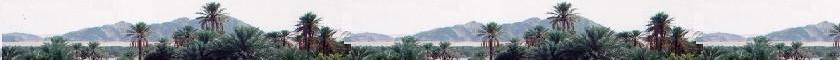Quantum Mechanics

Schrodinger equation

Quantum Mechanics

Propagators : Pg

Quantum Simple Harmonic
Oscillator QSHO

Quantum Mechanics
Simulation With GNU Octave

© The scientific sentence. 2010

# Dirac equation: free particle

### 1. Dirac's equation

The main idea is to change the D'Alembertian operator in Klein_Gordon equation into a Lorentz invariant.

Here is Klein-Gordon equation is:

(1/c2)∂2/∂t2ψ - ∇2ψ + (mo2c22)ψ = 0

or
☐ψ + (moc/ħ)2ψ = 0

where
☐ = (1/c2)∂2/∂t2 - ∇2

Like a 4-Vector, the wave function ψ(r,t) for which will be applied the square root of the D'Alembertian must have 4 components. Therefore, this square root of the D'Alembertian will be transformed by matrices.

Dirac factorised the d'Alembertian as follows:

2 = - (1/c2)∂2/∂t2
= (A∂/∂x + B∂/∂y + C∂/∂z + (i/c)D∂/∂t) (A∂/∂x + B∂/∂y + C∂/∂z + (i/c)D∂/∂t)

The cross-terms of the operator A, B, C, and D will vanish, and their square must be equal to the operator indentity I. That is:
Their anticommutators {X,Y} = 0 and X2 = Y2 = I. (X and Y are any operator A, B, C, or D).

Hence, the Klein_gordon equation:
☐ψ + (moc/ħ)2ψ = 0
becomes:

[∇2 - (1/c2)∂2/∂t2]ψ = (moc/ħ)2ψ
Or
(A∂/∂x + B∂/∂y + C∂/∂z + (i/c)D∂/∂t) ψ = (moc/ħ) ψ

Let's set:

A = iβα1, B = iβα2 , C = iβα3, and D = β

The above equation becomes:
iβ [(α1∂/∂x + α2∂/∂y + α3∂/∂z + (1/c)∂/∂t)] ψ = (moc/ħ) ψ

Using the symbol ∇ of the gradient operator with
the vector matrix α = (α1, α2, α3), we get:

iβ [α.∇ + (1/c)∂/∂t)] ψ = (moc/ħ) ψ

Multiplying the both sides by ħc, we get:

[iβħc α.∇ + iħ β ∂/∂t)] ψ = (moc2) ψ

Since β2 = 1, we have:
[iħc α.∇ + iħ∂/∂t)] ψ = (βmoc2) ψ

Using the quantum expression of momentum operator P = -iħ∇, we get:
[- c α.P + iħ∂/∂t)] ψ = (βmoc2) ψ

We find finally the equation:

(iħ∂/∂t) ψ = (cα.P + βmoc2) ψ

That is the Dirac equation.

Dirac equation:
(iħ∂/∂t) ψ = (c α.P + βmoc2) ψ

α1, α2, α3, and β are 4x4 matrices.

### 2. Dirac's matrices

Dirac defined the matrices α1, α2, α3, and β as the following: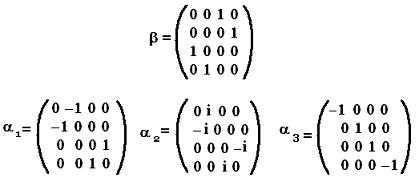### 2. γ-Dirac's matrices

More better, new matrices, known as the gamma matrices are defined:
γ0 = β
γi = β αi,
i = 1, 2, 3.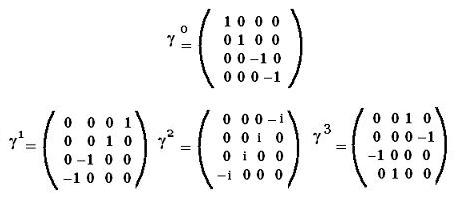Dirac Equation becomes;
(iħγ0∂/∂t) ψ = (c γ.P + moc2) ψ

(iħγ0∂/∂t) ψ = (c γ.P + moc2) ψ

Using the Pauli's matrices: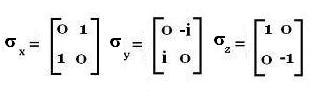we can write: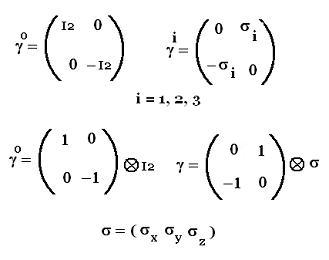chimie labs | Physics and Measurements | Probability & Statistics | Combinatorics - Probability | Chimie | Optics | contact |